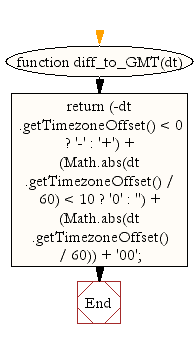# JavaScript: Get difference to Greenwich time in hours

## JavaScript Datetime: Exercise-39 with Solution

Write a JavaScript function to get difference to Greenwich time (GMT) in hours.

Test Data :
dt = new Date();
console.log(diff_to_GMT(dt));
"+05.500"

Sample Solution:-

HTML Code:

``````<!DOCTYPE html>
<html>
<head>
<meta charset="utf-8">
<title>JavaScript function to get difference to Greenwich time (GMT) in hours.</title>
</head>
<body>

</body>
</html>
```
```

JavaScript Code:

``````
function diff_to_GMT(dt)
{
return (-dt.getTimezoneOffset() < 0 ? '-' : '+') + (Math.abs(dt.getTimezoneOffset() / 60) < 10 ? '0' : '') + (Math.abs(dt.getTimezoneOffset() / 60)) + '00';
}

dt = new Date();
console.log(diff_to_GMT(dt));

dt = new Date(1989, 10, 1);
console.log(diff_to_GMT(dt));
```
```

Sample Output:

```+05.500
+05.500
```

Flowchart:Live Demo:

See the Pen JavaScript - Get difference to Greenwich time in hours-date-ex-39 by w3resource (@w3resource) on CodePen.

Improve this sample solution and post your code through Disqus

What is the difficulty level of this exercise?

﻿

## JavaScript: Tips of the Day

Convert tabs to spaces, where each tab corresponds to count spaces

Example:

```const tips_expandTabs = (str, count) => str.replace(/\t/g, ' '.repeat(count));
console.log(tips_expandTabs('\t\tw3resource', 4));
```

Output:

```"        w3resource"
```